# On the penalties for late assignments

Posted on Sun 21 April 2019 in opinion

Late submission of important assignments disrupts effective teaching. Students who fail to meet deadlines give themselves additional time with which to complete their assignment, thereby gaining an unfair advantage over their peers. Late submission also disrupts grading, imposing unfair burdens on graders and classmates. An important question which remains is how to best penalize late submissions. Many institutions choose either to assign a fixed penalty to all late assignments, or to allow full discretion to the course administrator. Both options are undesirable.

In this document we propose three principles for the penalization of late assignments and suggest an appropriate method for scoring late assignments. A practical algorithm and examples are provided.

Numerical examples and simulations are included below; the raw code for this notebook is available at https://github.com/jdossgollin/jdossgollin.github.io/tree/source/content/posts.

In :
import numpy as np
from scipy import stats
import matplotlib.pyplot as plt


# Development of Principles¶

We begin by laying out three principles which inform our approach.

## Principle 1: Transparency and Fairness¶

In a fair course, the characteristics of an individual student should have no bearing on the penalty their assignment earns for late submission. Any method which allows discrimination, implicit or explicit, based on age, race, gender, sexual orientation, or any other characteristic should be avoided. Instead, the lateness penalty should depend only upon the hours that the penalty is late.

## Principle 2: Decreasing Marginal Penalties¶

We assume decreasing marginal benefits of extra time. This implies that the difference in penalty between an assignment which is three hours late and one which is two hours late should be greater than the dfiference between an assignment turned in three days plus three hours late and one turned in three days plus two hours late.

## Principle 3: Aversion to Risk¶

A broad economic literature on risk aversion documents the preference of individuals to avoid outcomes which have highly uncertain negative penalties. This suggests that adding uncertainty to lateness penalties may discourage students from submitting late assignments without decreasing the size of the average (i.e., expected) penalty. Introducing noise also discourages students from deliberately turning assignments in late in order to gain a strategic advantage, as this decision must now account for uncertainty.

# Principled Approach¶

We propose a penalization approach which depends only on the time difference between the due date and submission, $x$ (Principle 1). We do this by imposing decreasing marginal penalties through a logistic function (Principle 2) whose parameters are randomly drawn (Principle 3).

## Functional Form¶

The 3-parameter logistic function is

$$f(x) = \frac{L}{1 + e^{k(x - x_0)}}$$

where $x_9$ is the $x$-value of the sigmoid's midpoint, $L$ is the curve's maximum value (i.e., the maximum penalty), and $k$ describes the steepness of the curve. We suggest using this equation as an additive penalty in order to conform with university regulations.

The following code produces the plot of a generic logistic function.

In :
def logistic(x, L, k, x0):
return L / (1 + np.exp(-k * (x - x0)))

x = np.linspace(-6, 6, 500)
y = logistic(x, L=1, x0=0, k=1)
plt.plot(x, y)
plt.title("A Generic Logistic Function: $x0=0, L=1, k=1$")
plt.grid()
plt.show()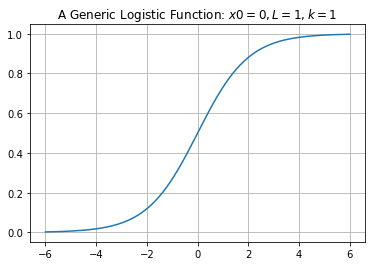## Parameter Selection¶

Implementing a logistic lateness penalty requires identifying reasonable values of $\{L, k, x_0\}$. Keeping in mind our decision to induce some randomness in the hyperparameters, we draw each from a distribution with fixed hyperparameters (discussed later).

We begin by considering the maximum penalty $L$. Since a penalty greater than 1 would imply that a perfect assignment earned a negative grade, and since a penalty lower than 0 would imply a reward for turning in an assignment late, we use the bounded Beta distribution $$L \sim \text{Beta}(\alpha_L, \beta_L).$$

We next consider the steepness coefficient $k$. Since a value of $k < 0$ would imply that the penalty decreases as lateness $x$ increases, we should bound $k > 0$. There is no justification for setting a lower bound on $k$. We therefore set $-k$ to follow a log-normal distribution: $$\log k \sim \mathcal{N}(\mu_k, \sigma_k^2)$$ where $\mathcal{N}(\mu, \sigma^2)$ defines a Normal distribution with mean $\mu$ and variance $\sigma^2$.

We finally consider the curve midpoint $x_0$. Based on Principle 2 we fix $x_0=0$. However, this induces a bias of $\frac{L}{2}$ in the penalty which must be accounted for. Instead, we add a fixed penalty $y_0$ for all late assignments. Bounding $y_0 > 0$, we again use the log-normal distributino $$\log y \sim \mathcal{N} (\mu_y, \sigma_y^2).$$

The final functional form is thus $$f(x) = y_0 + \frac{L}{1 + e^{-kx}} - \frac{L}{2}$$

We now turn to the question of selecting the hyperparameters $\{ \alpha_L, \beta_L, \mu_k, \sigma_k, \mu_y, \sigma_y \}$.

## Hyperparameter Selection for $L$¶

There is not a single correct value for the hyperparameters $\{\alpha_L, \beta_L \}$. However, we believe that a reasonable expectation for $L$ is 15\% (0.15) and a reasonable variance is 0.025. In order to choose $\alpha_L$ and $\beta_L$ we note that a Beta distribution has expectation $$\frac{\alpha}{\alpha+\beta}$$ and variance $$\frac{\alpha\beta}{(\alpha + \beta)^2 (1 + \alpha + \beta)}.$$ By choosing a mean and variance for $L$, it is straightforward to calculate $\alpha_L$ and $\beta_L$. The following codes return the corresponding values of $\alpha_L$ and $\beta_L$ with a mean of 0.15 (15 percent) and a standard deviation of 2.5 percent.

In :
def calc_alpha_beta(expectation, variance):
aplusb = (expectation * (1 - expectation)) / variance - 1
alpha = (aplusb) * expectation
beta = (aplusb) * (1 - expectation)
return alpha, beta

alpha_L, beta_L = calc_alpha_beta(expectation=0.15, variance=(0.025 ** 2))
print(f"alpha_L: {alpha_L:f}")
print(f"beta_L: {beta_L:f}")

alpha_L: 30.450000
beta_L: 172.550000


We can plot the distribution of $L$ using these parameters (recall that 1.0 is 100 points)

In :
x = np.linspace(0, 1, 500)
y = stats.beta.pdf(a=alpha_L, b=beta_L, x=x)
plt.plot(x, y)
plt.xlabel("Value of $L$")
plt.ylabel("Probability Density")
plt.title("Probability Density Function for $L$")
plt.yticks([])
plt.show()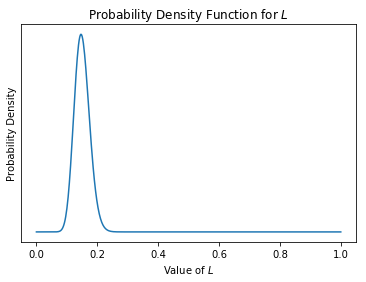## Hyperparameter Selection for $y_0$¶

As with $L$, there is no correct value. However, a penalty around 4 points seems reasonable. We suggest that the values $\mu_y = \log(0.04)$ and $\sigma_y = 0.2$ seem reasonable.

In :
mu_y = np.log(0.04)
sigma_y = 0.2
x = np.linspace(0, 0.15, 500)
y = stats.norm.pdf(x=np.log(x), loc=mu_y, scale=sigma_y)
plt.plot(x, y)
plt.xlabel("Value of $y_0$")
plt.ylabel("Probability Density")
plt.title("Probability Density Function for $y_0$")
plt.yticks([])
plt.show()

/usr/local/miniconda3/envs/jdossgollin/lib/python3.7/site-packages/ipykernel_launcher.py:4: RuntimeWarning: divide by zero encountered in log
after removing the cwd from sys.path.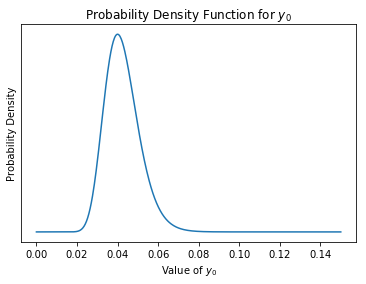## Hyperparameters for $k$¶

Next we consider the hyperparameters $\{ \mu_k, \sigma_k \}$. Let us assume that the units for $x$ are hours. We will set $\mu_k = \log (0.1)$ and $\sigma_k = 0.075$.

In :
mu_k = np.log(0.1)
sigma_k = 0.075
x = np.linspace(0.075, 0.15, 500)
y = stats.norm.pdf(x=np.log(x), loc=mu_k, scale=sigma_k)
plt.plot(x, y)
plt.xlabel("Value of $k$")
plt.ylabel("Probability Density")
plt.title("Probability Density Function for $k$")
plt.yticks([])
plt.show()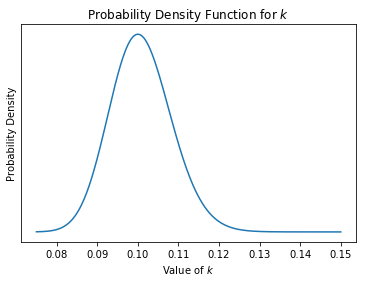## Distribution of $f(x)$¶

Ultimately the quantity of concern is not the individual parameters but the penalty. To explore the penalty as a function of tardiness, $x$, we simulate 10000 late submissions with different values of $x$ and for each submission draw each parameter individually and then calculate the penalty.

In :
def get_one_penalty(x, alpha_L, beta_L, mu_y, sigma_y, mu_k,  sigma_k):
L = np.random.beta(a=alpha_L, b=beta_L)
y0 = np.exp(np.random.normal(loc=mu_y, scale=sigma_y))
k = np.exp(np.random.normal(loc=mu_k, scale=sigma_k))
penalty = y0 - (L / 2.0) + L / (1.0 + np.exp(-k * x))
return penalty

In :
def get_many_penalty(x, alpha_L, beta_L, mu_y, sigma_y, mu_k,  sigma_k):
return np.array([
get_one_penalty(xi, alpha_L, beta_L, mu_y, sigma_y, mu_k,  sigma_k)
for xi in x])

In :
x = np.random.uniform(low=0, high=100, size=10000)
y = get_many_penalty(x, alpha_L=alpha_L, beta_L=beta_L, mu_y=mu_y, sigma_y=sigma_y, mu_k=mu_k, sigma_k=sigma_k)

In :
plt.figure(figsize=(10, 7))
plt.scatter(x, y, alpha=0.1)
plt.xlabel("Hours Late")
plt.ylabel("Size of Penalty [Porportion]")
plt.title("Simulated Penalties Awarded")
plt.show()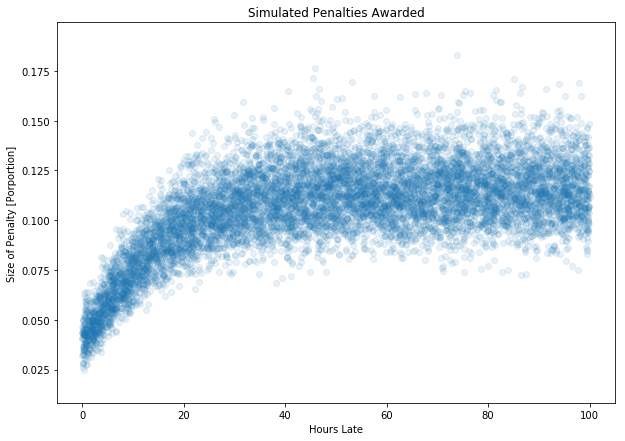# Conclusions¶

This approach meets the criteria of being

1. fair, in the sense that lateness penalties depend only on the time of submission;
2. decreasing marginal penalties; and
3. uncertain penalties.

The figure shown above shows that both the expected penalty and the variance of the penalty increase as $x$ increases, but both plateau after a few days. The specific hyperparameters used here can be easily adapted to the preferences of individual instructors. Note that implementation of this algorithm will require a transparent metric for setting the seed of the computer's random number generator.